# Solving & Graphing Quadratics Chapter Exam

Exam Instructions:

Choose your answers to the questions and click 'Next' to see the next set of questions. You can skip questions if you would like and come back to them later with the yellow "Go To First Skipped Question" button. When you have completed the practice exam, a green submit button will appear. Click it to see your results. Good luck!

Answered 0 of 30

### Page 1

#### Question 1 1. Rewrite the following equation in the vertex form.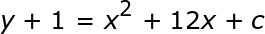#### Question 2 2. If the equation below told you a ball was 'h' feet in the air after 't' seconds, what would be the first step to finding out when it hit the ground?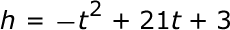#### Question 3 3. Solve.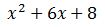#### Question 5 5. Solve.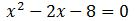### Page 2

#### Question 6 6. Use the quadratic formula to find the solutions to the equation below.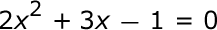#### Question 7 7. Rewrite the following equation in the vertex form.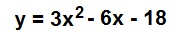#### Question 10 10. Rewrite the quadratic below in standard form: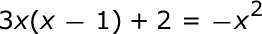### Page 3

#### Question 11 11. What is the b-value of the quadratic equation below?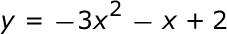#### Question 12 12. When completing the square on the quadratic equation listed below, how is c calculated?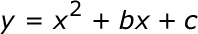#### Question 13 13. Which of the following are the solutions to the equation below?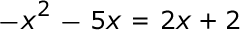#### Question 14 14. Solve.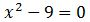#### Question 15 15. Solve.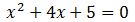### Page 4

#### Question 16 16. In order to complete the square on the following equation, what value would you add to both sides?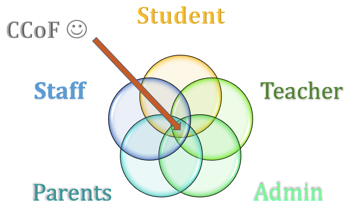#### Question 17 17. What is the solution to the following quadratic equation?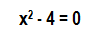#### Question 18 18. Which statement is true about the inequality below?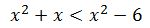#### Question 19 19. Expressing the equation below in the vertex form will give a value of 'h' = _____ .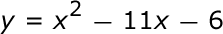### Page 5

#### Question 21 21. Solve.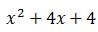#### Question 23 23. What would the first step be in completing the square of the following equation?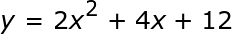#### Question 24 24. Solve the following inequality.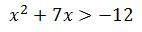#### Question 25 25. Factor.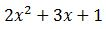### Page 6

#### Question 28 28. Solve the following quadratic equation by graphing: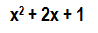#### Solving & Graphing Quadratics Chapter Exam Instructions

Choose your answers to the questions and click 'Next' to see the next set of questions. You can skip questions if you would like and come back to them later with the yellow "Go To First Skipped Question" button. When you have completed the practice exam, a green submit button will appear. Click it to see your results. Good luck!

Support